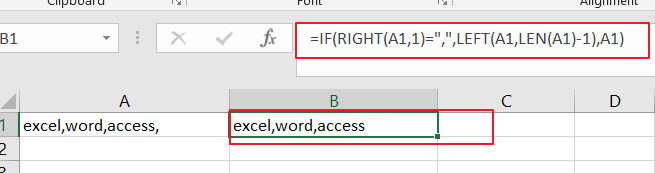# How to Remove Last or Trailing Commas in Excel

This post will guide you how to remove trailing commas from cell in Excel. How do I remove comma character at the end of a cell with a formula in Excel 2013/2016.

## Remove Trailing Commas

if you want to remove trailing commas from you Excel cells, and you can create a formula to check for a comma at the end of a string and then removes the final comma character. you can use the IF function, the RIGHT function, the LEFT function and the LEN function to remove the commas from each cell, like this:

=IF(RIGHT(A1,1)=”,”,LEFT(A1,LEN(A1)-1),A1)

You need to type this formula into a blank cell and press Enter key on your keyboard, and then drag the AutoFill handle over to the range of cells that you want to apply this formula.### Related Functions

• Excel IF function
The Excel IF function perform a logical test to return one value if the condition is TRUE and return another value if the condition is FALSE. The IF function is a build-in function in Microsoft Excel and it is categorized as a Logical Function.The syntax of the IF function is as below:= IF (condition, [true_value], [false_value])….
• Excel LEFT function
The Excel LEFT function returns a substring (a specified number of the characters) from a text string, starting from the leftmost character.The LEFT function is a build-in function in Microsoft Excel and it is categorized as a Text Function.The syntax of the LEFT function is as below:= LEFT(text,[num_chars])…t)…
• Excel RIGHT function
The Excel RIGHT function returns a substring (a specified number of the characters) from a text string, starting from the rightmost character.The syntax of the RIGHT function is as below:= RIGHT (text,[num_chars])…
• Excel LEN function
The Excel LEN function returns the length of a text string (the number of characters in a text string).The syntax of the LEN function is as below:= LEN(text)…
Related Posts
How to Split Cells by the First Space in Texts in Excel

Sometimes we may use space to separate texts to different groups in one cell, if we want to split this cell to multiple columns refer to space, we can implement this via ‘Text to Columns’ feature. But is there any ...

How to Convert Uppercase to Lowercase Except the First Letter in Excel

In excel, words can be entered in uppercase, lowercase or mixed. If we want to convert all uppercase to lowercase (except the first letter in some situations), we can use formula with some letter convert related functions to convert them ...

How to Convert Text to Time in Excel

This post will guide you how to convert time string to time in Excel. Assuming that you have a list of text string in your worksheet, and you wish to convert these time string to standard time format, how to ...

How to Convert mmddyy to Date in Excel

This post will guide you how to convert non-standard date formats or text to a standard date in Excel. If you have a date with mmddyy format, and you want to change it as the normal date format as mm/dd/yyyy ...

How to Convert Military Time to Standard Time in Excel

This post will guide you how to convert military time to standard time with a formula in Excel. How do I convert from military time 17:29:30 to regular time 5:29:30PM in Excel.  How do I Convert Standard time to military ...

How to Sort Data by Last Character in Excel

This post will guide you how to sort cells by last character in Excel. How do I sort data by the last character with a formula in Excel. How to sort cells based on the last character with User Defined ...

How to Remove the First/Last Word from Text string in Cell

This post will guide you how to remove the first and the last word from a text string in cells in Excel. How do I use a formula to remove first and last word of a text string in Excel. ...

How to Remove Prefix and Suffix in Given Cells in Excel

This post will guide you how to remove suffix form a range of cells in Excel. How do I remove prefix from text string in cells with a formula in Excel. Remove Prefix of Cells Remove Suffix of Cells Assuming ...